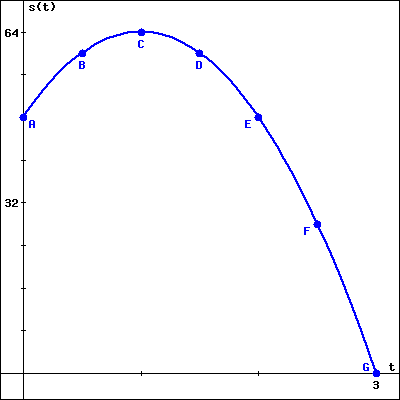Question
3 views

Suppose that the height s of a ball (in feet) at time t (in seconds) is given by the formula s(t)=64−16(t−1)^2. This function is graphed below on the interval 0≤t≤3.

When (in seconds after being released) did the ball reach its highest point?

How long (in seconds) after being released did the ball hit the ground?help_outlineImage Transcriptioncloses(t 64 C в D A E 32 F Gt fullscreen
check_circle

Step 1

Given a graph that represent the path of a ball. Height is defined with the help of function s(t) at time t is :

Step 2

First part:

Calculate the time(in seconds) when ball reach the highest point.

Here path of the ball is like a downward parabola.

Maximum height of the ball is defined in terms of the vertex of the parabola. SO, we will find the vertex of the parabola.

Step 3

In order to find the vertex of the parabola, we write the given function in form of vertex form of parabola.

The standard vertex form of parabola f(x) is : ...

### Want to see the full answer?

See Solution

#### Want to see this answer and more?

Solutions are written by subject experts who are available 24/7. Questions are typically answered within 1 hour.*

See Solution
*Response times may vary by subject and question.
Tagged in

### Algebra